# Force

A stock undoes acceleration if it is acted upon by another stock, by itself, or by an exogenous cause.

Consider the case where a stock x is influenced by another stock y. x and y can represent any variables, such as population numbers or social variables. The system dynamics diagram and differential equation are: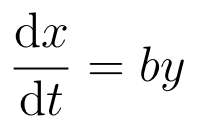where b is a constant.

Changes in y are reflected in changes in x, i.e. y is the cause of changes in x’s behaviour. This can be interpreted as y exerting a force on x as changes in y cause x to accelerate. Differentiate the equation: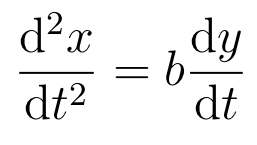If y is changing, non-zero derivative, then x accelerates. The influence from y causes x’s behaviour to deviate from uniform motion, the classic Newtonian definition of force. The value of the force is F = dy/dt.

The parameter b governs the response of x to y. The larger the value of b the more x responds to y. Thus b is the equivalent of the inverse of the mass, b = 1/m, as a lighter stock x responds more to a given force. Thus:is the equivalent of Newton’s second law of motion for system dynamics. The mass of the stock m converts the force from y into acceleration of x.

### Constant Force

Let the rate of change of y, its flow, be constant k: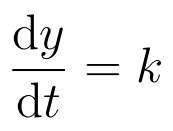Then the change in y causes x’s behaviour to deviate from a straight line, i.e. y induces

acceleration in x: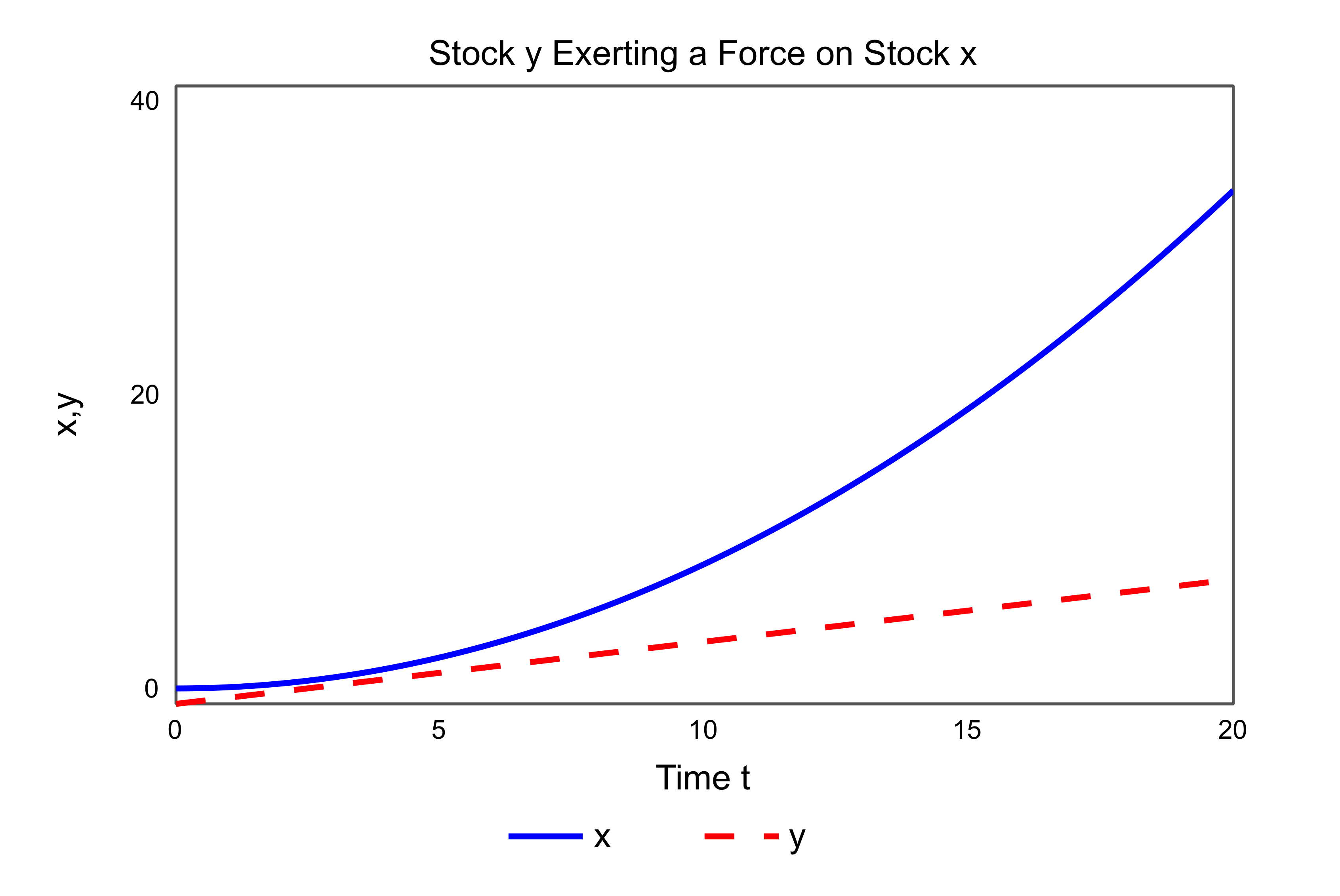Mathematically: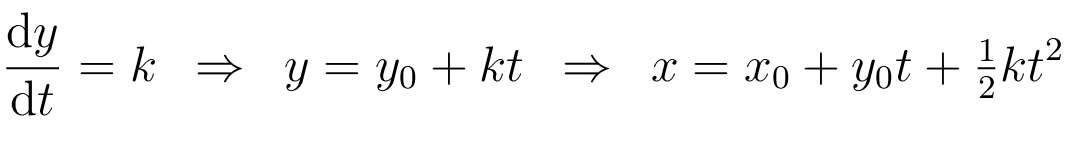Thus x is quadratic and its graph over time is curved.

If y is constant then k = 0 and thus x changes linearly, uniform motion. This is the system dynamics equivalent of Newton’s first law of motion. In this case a stock will remain constant, or change uniformly, unless acted upon by a force from another stock, itself, or an exogenous cause.

The impact of the constant force of y on x is: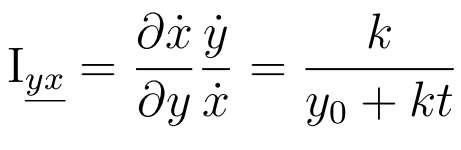Impact is a ratio measure of force, the acceleration of x imparted by y divided by the rate of change of x. It is also the force from y divided by the momentum of x. In the case of a constant force,  the impact of y is decreasing with time, showing that a constant force  has diminishing effect on stock as it increases, i.e. the time graph of the stock is becoming less curved.